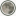My Shopping Cart Your shopping cart is empty Display Cart

Call us at +1 586 62 ELLIE / +1 586 62 35543# LinearRange object

Prototype for LinearRange objects

## Syntax

To create an empty LinearRange:

var linear_range = new LinearRange();

To create a LinearRange contains integers from start to start+length excluded (i.e. [start, start+length) integers):

var linear_range = new LinearRange(start, length);

To create a copy of a LinearRange:

var linear_range2 = new LinearRange (linear_range1);

## Parameters

start, length. Integers. start and length of the range to create
linear_range1. LinearRange object. Range to copy.

none.

ECMerge 2.1

## Description

LinearRange objects represents an interval in integers' space for which you provide a start and a length. It lets you compute intersections, containment tests and other useful operations.
There is no order enforced on the start and end of the LinearRange (i.e. length may be negative) but a function order() is provided to have start and length always such that start<=end if it is necessary for your needs.

## Properties

 Property Description is_empty Boolean. Read-Only. True if this range is empty (i.e. length == 0). start Integer. Start of the range. Modifying start also modifies end, keeping length untouched, such that end = length + start. end Integer. End of the range. Modifyng end also modifies length, such that length = end - start length Integer. Length of the range. Modifyng length also modifies end, such that end = length + start

## Examples

### Example 1.

Creating a LinearRange containing integers from 5 to 9 (excluded).

var linear_range = new LinearRange (5, 4);

none.## Method contains

### Syntax

function contains (range)
function contains (start, length)
returns a Boolean

### Parameters

range. LinearRange object. Range that we test to know if it is contained by this object.
start, lengh. Integers. Start and length as if contains (LinearRange (start, length)) was called.

### Method Of

LinearRange object.

ECMerge 2.1

### Description

Returns true if the tested range is actually contained in this object. A range is considered contained inside another object:

• if its length is 0, then range.start should be within [start, end]
• if its length is not 0, then if all the elements in range are also included in this object.

### Examples

#### Example 1.

Logs whether a range is contained by another (true in this case):

log (LinearRange (100, 10).contains (LinearRange (105, 5)));

LinearRange object## Method empty

### Syntax

function empty ( )

none.

### Method Of

LinearRange object

ECMerge 2.1

### Description

Sets length and start to 0 (zero).

LinearRange object## Method enlarge_to_contain

### Syntax

function enlarge_to_contain (range)
function enlarge_to_contain (start, length)

### Parameters

range. LinearRange object. Range that we to include in this object.
start, lengh. Integers. Start and length as if enlarge_to_contain (LinearRange (start, length)) was called.

### Method Of

LinearRange object.

ECMerge 2.1

### Description

Modifies this object such that all the integers in range are also included in this object.

### Examples

#### Example 1.

Enlarges a range with another then logs the result:

var range = LinearRange (25, 50);
range.enlarge_to_contain (23, 40);
log ("start=" + range.start +", length=" + range.length);

which prints:

start=23, length=52

LinearRange object## Method intersection

### Syntax

function intersection (range)
returns a LinearRange object

### Parameters

range. LinearRange object. Range for which we want to compute the intersection with this object.

### Method Of

LinearRange object.

ECMerge 2.1

### Description

Computes the intersection between this object and range and returns the result as a LinearRange object.

### Examples

#### Example 1.

Intersects a range with another then logs the result:

var range = LinearRange (25, 50).intersection (LinearRange (23, 40));
log ("start=" + range.start +", length=" + range.length);

which prints:

start=25, length=38

LinearRange object## Method order

### Syntax

function order ( )

none.

### Method Of

LinearRange object.

ECMerge 2.1

### Description

Ensures that start is before or equal to end (and as such that length is positive or zero).

### Examples

#### Example 1.

Re-orders a range with negative length then logs the result:

var range = LinearRange (25, -50);
ranger.order ();
log ("start=" + range.start +", length=" + range.length);

which prints:

start=-25, length=50